Module 24 - Power Series Introduction | Lesson 1 | Lesson 2 | Lesson 3 | Self-Test Lesson 24.3: Taylor Series In Lesson 24.2 you found Maclaurin series that approximate functions near x = 0. This lesson investigates how to find a series that approximates a function near x = a, where a is any real number. A Taylor Series Given a function f that has all its higher order derivatives, the series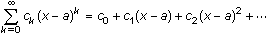, where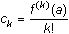is called the Taylor series for f centered at a. The Taylor series is a power series that approximates the function f near x = a. The partial sum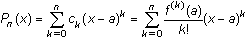is called the nth-order Taylor polynomial for f centered at a. Every Maclaurin series, including those studied in Lesson 24.2, is a Taylor series centered at zero. The Taylor Polynomial of ex Centered at 1 The second-order Taylor polynomial centered at 1 for the function f(x) = ex can be found by using a procedure similar to the procedure given in Lesson 24.2. The coefficient of the term (x - 1)k in the Taylor polynomial is given by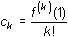. This formula is very similar to the formula for finding the coefficient of xk in a Maclaurin polynomial where the derivative is evaluated at 0. In this Taylor polynomial, the derivative is evaluated at 1, the center of the series. The coefficients of the second-order Taylor polynomial centered at 1 for ex are f(1) = e f '(1) = e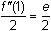So the second-order Taylor polynomial for ex centered at 1 is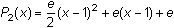, and near x = 1, exP2(x). The Taylor series for ex centered at 1 is similar to the Maclaurin series for ex found in Lesson 24.2. However, the terms in the Taylor series have powers of (x - 1) rather than powers of x and the coefficients contain the values of the derivatives evaluated at x = 1 rather than evaluated at x = 0. Graphing the function and the polynomial illustrate that the polynomial is a good approximation near x = 1. Graph y = ex and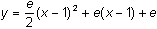in a [-2, 3, 1] x [-3, 10, 1] window.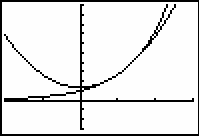The second-order Maclaurin polynomial you found in Lesson 24.2,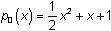, is tangent to f(x) = ex at x = 0 and has the same concavity as f(x) = ex at that point. The polynomial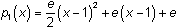, which is centered at x = 1, is tangent to f(x) = ex at x = 1 and has the same concavity as f(x) = ex at that point. 24.3.1 Find the second-order Taylor polynomial centered at 1 for the function f(x) = ln x. Graph this polynomial together with f(x) = ln x. Click here for the answer. Other Taylor Series The Taylor series for one function can be used to find a Taylor series for a related function. The third-order Taylor polynomial centered at 1 for f(x) = ln x is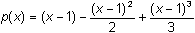. The derivative of f(x) = ln x is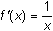. The derivative of p(x) gives the second-order Taylor polynomial for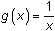centered at 1. 24.3.2 Find the second-order Taylor polynomial forcentered at 1 using the derivative of p(x) and graph it with. Click here for the answer. The Taylor Series for cos x2 Other modifications of a Taylor series yield other Taylor-series. For example, replacing each x with x2 in the Taylor series for f(x) = cos(x) gives the Taylor series for g(x) = f(x2) = cos(x2).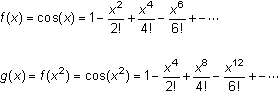Graph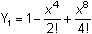and Y2 = cos(x2) in a [-2,2,1] x [-2,2,1] window.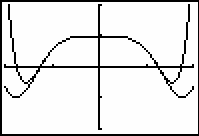< Back | Next > ©Copyright 2007 All rights reserved. | Trademarks | Privacy Policy | Link Policy